Test: Parallel Lines And Transversals

# Test: Parallel Lines And Transversals - Class 9

Test Description

## 15 Questions MCQ Test Mathematics (Maths) Class 9 - Test: Parallel Lines And Transversals

Test: Parallel Lines And Transversals for Class 9 2023 is part of Mathematics (Maths) Class 9 preparation. The Test: Parallel Lines And Transversals questions and answers have been prepared according to the Class 9 exam syllabus.The Test: Parallel Lines And Transversals MCQs are made for Class 9 2023 Exam. Find important definitions, questions, notes, meanings, examples, exercises, MCQs and online tests for Test: Parallel Lines And Transversals below.
Solutions of Test: Parallel Lines And Transversals questions in English are available as part of our Mathematics (Maths) Class 9 for Class 9 & Test: Parallel Lines And Transversals solutions in Hindi for Mathematics (Maths) Class 9 course. Download more important topics, notes, lectures and mock test series for Class 9 Exam by signing up for free. Attempt Test: Parallel Lines And Transversals | 15 questions in 15 minutes | Mock test for Class 9 preparation | Free important questions MCQ to study Mathematics (Maths) Class 9 for Class 9 Exam | Download free PDF with solutions
 1 Crore+ students have signed up on EduRev. Have you?
Test: Parallel Lines And Transversals - Question 1

### Line A makes an angle of 30 degrees with the line B, also line C makes an angle of 30 degrees at the other end with line B on the opposite side. Then, ______.

Test: Parallel Lines And Transversals - Question 2

### In the figure below, the angle a = 150°. The other angle in the figure which is also 150° is: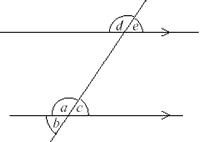Detailed Solution for Test: Parallel Lines And Transversals - Question 2

Explanation : Angle d is corresponding angle to a

so , if a = 150o

then d is also equal to 150o

Test: Parallel Lines And Transversals - Question 3

### If a transversal intersects two parallel lines, then each pair of corresponding angles is ………

Detailed Solution for Test: Parallel Lines And Transversals - Question 3
By using corresponding angle axiom to find out the relation between the alternate interior angle when a transversal two parallel lines .In fig transversal PS intersect parallel lines AB and CD at points Q and R . angle BQR = Angle QRC andAQR = angle QRD we know that angle PQA = angle QRC ……1corresponding angle axiom angle PQA = angle BQR now , from 1 and 2 you may conclude angle BQR= angle QRCsimilarly,. angle AQR= angle QRD
Test: Parallel Lines And Transversals - Question 4

In the figure, PQ || MN, the value of x will be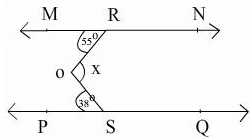Detailed Solution for Test: Parallel Lines And Transversals - Question 4
First Mr parallel EF and RO is transversal line angle MRO=angle ROF=(alt.int .angle)toh angle MRO=55 degree and angle ROF =angle 1 toh 55=angle 1 is angle 1 =55 then second EF parallel PQ and OS is transversal line toh angle 2 = angle OSP is = (apt.int angle)toh angle 2 = 38 degree and Angle ROS = x hoga woo toh jnte hoge aap log toh x= angle 1 +angle 2 is toh angle 1hoga 55 or angle 2 hoga 38 toh x = 93
Test: Parallel Lines And Transversals - Question 5

The figure below shows a parallelogram. What is the measure of ∠b?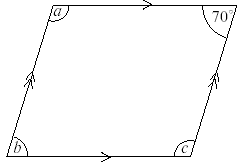Test: Parallel Lines And Transversals - Question 6

In the figure if l║m, n║p and ∠1 = 85°, then ∠2 is equal to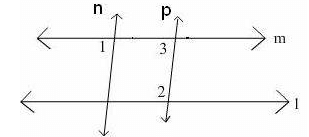Test: Parallel Lines And Transversals - Question 7

In the given figure, PQ||RS and EF||QS. If ∠PQS = 60°, then the measure of ∠RFE is: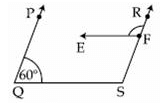Detailed Solution for Test: Parallel Lines And Transversals - Question 7

60°+ QSR=180°[co interior angles]

QSR=120°

RFE=QSR

So,RFE=120°

Test: Parallel Lines And Transversals - Question 8

In the above figure AB || CD. Also, ∠EAB = 84° and ∠ECD = 126°. Then, the value of “x” is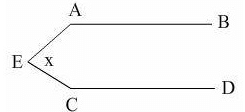Test: Parallel Lines And Transversals - Question 9

Observe the given figure and choose the correct statement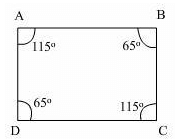Detailed Solution for Test: Parallel Lines And Transversals - Question 9
IN THIS CORRECT ANSWER IS D . BECAUSE IF WE CONSIDERED AB AND CD AS 2 LINE AND BC AND AD AS TWO TRANSVERSAL . THEN ANGLE 'B' = 65 AND ANGLE C = 115 THEN ANGLE B + C = 180 DEGREE BUT THEY ARE ANGLES ON THE SAME SIDE AND THEY ARE SUPPLYMENTRY BUT THIS ONLY HAPPENS IN A PARRALLEL LINES THERE AB || CD .
Test: Parallel Lines And Transversals - Question 10

In the figure, AB║CD. If ∠2 = (2x + 30)°, ∠4 = (x + 2y)° and ∠6 = (3y+10)° the measure of ∠5 is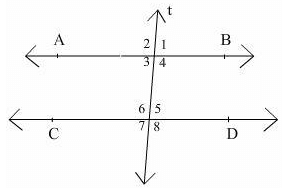Test: Parallel Lines And Transversals - Question 11

In fig., if m||n and ∠a : ∠b = 2 : 3, the measure of ∠h is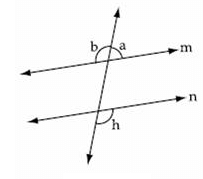Test: Parallel Lines And Transversals - Question 12

In the figure, AB || CD and ∠F = 30° the value of ∠ECD is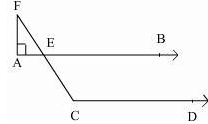Detailed Solution for Test: Parallel Lines And Transversals - Question 12
In triangle AFE A+F+E=180 (angle sum property of triangle) 90+30+e=180 e=60 fea=bec=60 bec+dec=180. (co interior) dec=120degree.
Test: Parallel Lines And Transversals - Question 13

If two parallel lines are intersected by a transversal then, pair of alternate interior angles are:

Test: Parallel Lines And Transversals - Question 14

In the adjoining figure, ABCD is a parallelogram in which ∠A = 72°. Calculate ∠B, ∠C and ∠D.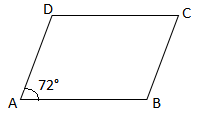Detailed Solution for Test: Parallel Lines And Transversals - Question 14

ABCD is a parallelogram and ∠ A = 72°

We know that opposite angles of a parallelogram are equal

∴ ∠A = ∠C and ∠B = ∠D

∴ ∠C = 72°

∠A and ∠B are adjacent angles

i.e., ∠A + ∠B = 180°

⇒ ∠B = 180° – ∠A

⇒∠B = 180° – 72° = 108°

∠B = ∠D =108°

Hence, ∠B = ∠D = 108° and ∠C = 72°

Test: Parallel Lines And Transversals - Question 15

Two parallel lines l and m are such that A, C are the points on l and B, D are the points on m. AB and CD are perpendicular to both l and m. If AB = 4 cm, then CD =

## Mathematics (Maths) Class 9

42 videos|378 docs|65 tests
Information about Test: Parallel Lines And Transversals Page
In this test you can find the Exam questions for Test: Parallel Lines And Transversals solved & explained in the simplest way possible. Besides giving Questions and answers for Test: Parallel Lines And Transversals, EduRev gives you an ample number of Online tests for practice

## Mathematics (Maths) Class 9

42 videos|378 docs|65 tests